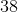# ISEE Upper Level Math : Other Factors / Multiples

## Example Questions

← Previous 1

### Example Question #1 : How To Factor A Number

What is the product of all of the factors of 25?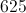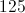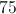Explanation:

25 has three factors: 1, 5, and 25. Their product is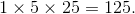### Example Question #1 : How To Factor A Number

Which of these numbers has exactly three factors?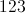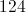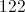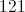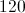Explanation:

None of the choices are prime, so each has at least three factors. The question, then, is which one has only three factors?

We can eliminate four choices by showing that each has at least four factors - that is, at least two different factors other than 1 and itself: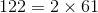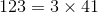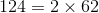Each, therefore, has at least four factors.

However, the only way to factor 121 other thanis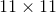. Therefore, 121 has only 1, 11, and 121 as factors, and it is the correct choice.

### Example Question #11 : Numbers And Operations

What is the sum of all of the factors of 60?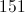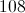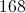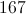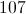Explanation:

60 has twelve factors: 1, 2, 3, 4, 5, 6, 10, 12, 15, 20, 30, and 60.

Their sum is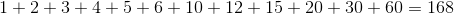.

### Example Question #11 : Numbers And Operations

Give the prime factorization of 135.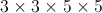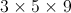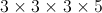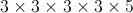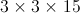Explanation: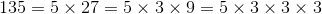3 and 5 are both primes, so this is as far as we can go. Rearranging, the prime factorization is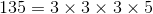.

### Example Question #1 : How To Factor A Number

Which of the following digits can go into the box to form a three-digit number divisible by 3?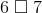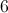Explanation:

Place each of these digits into the box in turn. Divide each of the numbers formed and see which quotient yields a zero remainder: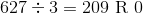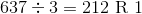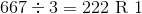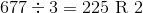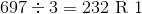Only 627 is divisible by 3 so the correct choice is 2.

### Example Question #451 : Isee Upper Level (Grades 9 12) Mathematics Achievement

Which of the following digits can go into the box to form a three-digit number divisible by 4?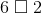None of the other choices is correct.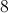None of the other choices is correct.

Explanation:

For a number to be divisible by 4, the last two digits must form an integer divisible by 4. 2 (02), 22, 62, and 82 all yield remainders of 2 when divided by 4, so none of these alternatives make the number a multiple of 4.

### Example Question #1 : Other Factors / Multiples

Which of the following is divisible by?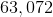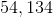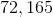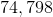Explanation:

Numbers that are divisble by 6 are also divisble by 2 and 3. Only even numbers are divisible by 2, therefore, 72165 is excluded. The sum of the digits of numbers divisible by 3 are also divisible by 3. For example,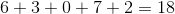Because 18 is divisible by 3, 63,072 is divisible by 3.

### Example Question #12 : Numbers And Operations

Letbe the set of all integerssuch thatis divisible byand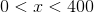. How many elements are in?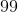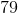Explanation:

The elements are as follows: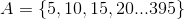This can be rewritten as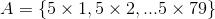.

Therefore, there areelements in.

### Example Question #2 : How To Factor A Number

Letbe the set of all integerssuch thatis divisible by three and. How many elements are in?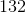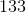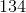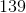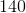Explanation:

The elements are as follows: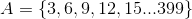This can be rewritten as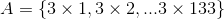.

Therefore, there areelements in.

### Example Question #2 : How To Factor A Number